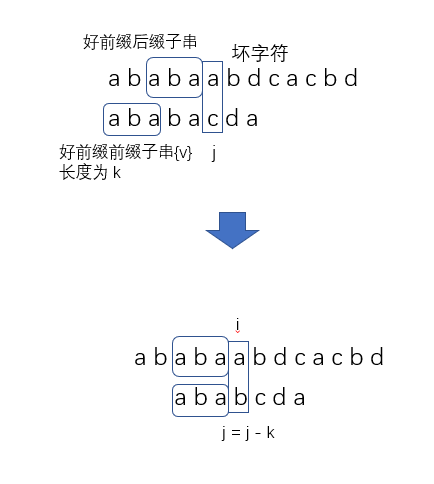## KMP 算法原理

KMP 算法全称 Knuth-Morris-Pratt 字符串查找算法， 由Donald Knuth、Vaughan Pratt、James H. Morris三人于1977年联合发表，故取这3人的姓氏命名此算法。KMP 算法就是在寻找这样一种规律: 在模式串和主串匹配的过程中，如果遇到坏字符，如何根据已匹配的前缀向后滑动最多的位数？a0-1next = -1
ab1-1next = -1
aba20next = 0
abab31next = 1
ababc4-1next = -1

int KMP::find(const string& main_string)
{
int match = 0; // 好前缀的长度

for (auto i = 0; i < main_string.length(); i++)
{
while (match > 0 && main_string[i] != ptrn[match])
match = next[match - 1] + 1;

if (main_string[i] == ptrn[match])
match++;

// 找到匹配的字符串
if (match == ptrn.length())
return i - match + 1;
}

return -1;
}

## 失效函数的计算方法

KMP 使用的方法非常巧妙，用到了一些动态规划的思想。下面我们就来看看它是怎么实现高效构建 next 数组的。

void KMP::generate_next()
{
next = -1;

int k = -1; // 最长可匹配前缀子串的结束下标

for (auto i = 1; i < ptrn.length(); i++)
{
// 寻找次长可匹配后缀子串
while (k != -1 && ptrn[k + 1] != ptrn[i])
k = next[k];

if (ptrn[k + 1] == ptrn[i])
k++;

next[i] = k;
}
}

## KMP算法 C++ 实现

KMP.h

#pragma once

#include <string>
#include <vector>

using std::string;
using std::vector;

class KMP
{
private:
string ptrn;
vector<int> next;

void generate_next();

public:
KMP();
explicit KMP(const string& p);
~KMP();

bool set_pattern(const string& p);

int find(const string& main_string);
};

KMP.cpp

#include "KMP.h"

void KMP::generate_next()
{
next = -1;

int k = -1; // 最长可匹配前缀子串的结束下标

for (auto i = 1; i < ptrn.length(); i++)
{
// 寻找次长可匹配后缀子串
while (k != -1 && ptrn[k + 1] != ptrn[i])
k = next[k];

if (ptrn[k + 1] == ptrn[i])
k++;

next[i] = k;
}
}

KMP::KMP()
= default;

KMP::KMP(const string& p)
{
set_pattern(p);
}

KMP::~KMP()
= default;

bool KMP::set_pattern(const string& p)
{
ptrn = p;
next.resize(ptrn.length());

generate_next();

return true;
}

int KMP::find(const string& main_string)
{
int match = 0; // 好前缀的长度

for (auto i = 0; i < main_string.length(); i++)
{
while (match > 0 && main_string[i] != ptrn[match])
match = next[match - 1] + 1;

if (main_string[i] == ptrn[match])
match++;

// 找到匹配的字符串
if (match == ptrn.length())
return i - match + 1;
}

return -1;
}

#include <iostream>
#include <chrono>
#include "KMP.h"
using namespace std;
using namespace chrono;

int main()
{
string a, b;

while (cin >> a >> b)
{
auto begin = system_clock::now();

KMP kmp(b);
int pos = kmp.find(a);

auto end = system_clock::now();
auto duration = duration_cast<microseconds>(end - begin);

cout << "查找结果为: " << pos << endl;
cout << "一共花费了: " << double(duration.count()) * \
microseconds::period::num / microseconds::period::den \
<< "秒" << endl;
}

return 0;
}
Last modification：October 17th, 2019 at 03:58 pm
If you think my article is useful to you, please feel free to appreciate